# 15.9: Lewis Acids and Bases

$$\newcommand{\vecs}{\overset { \rightharpoonup} {\mathbf{#1}} }$$ $$\newcommand{\vecd}{\overset{-\!-\!\rightharpoonup}{\vphantom{a}\smash {#1}}}$$$$\newcommand{\id}{\mathrm{id}}$$ $$\newcommand{\Span}{\mathrm{span}}$$ $$\newcommand{\kernel}{\mathrm{null}\,}$$ $$\newcommand{\range}{\mathrm{range}\,}$$ $$\newcommand{\RealPart}{\mathrm{Re}}$$ $$\newcommand{\ImaginaryPart}{\mathrm{Im}}$$ $$\newcommand{\Argument}{\mathrm{Arg}}$$ $$\newcommand{\norm}{\| #1 \|}$$ $$\newcommand{\inner}{\langle #1, #2 \rangle}$$ $$\newcommand{\Span}{\mathrm{span}}$$ $$\newcommand{\id}{\mathrm{id}}$$ $$\newcommand{\Span}{\mathrm{span}}$$ $$\newcommand{\kernel}{\mathrm{null}\,}$$ $$\newcommand{\range}{\mathrm{range}\,}$$ $$\newcommand{\RealPart}{\mathrm{Re}}$$ $$\newcommand{\ImaginaryPart}{\mathrm{Im}}$$ $$\newcommand{\Argument}{\mathrm{Arg}}$$ $$\newcommand{\norm}{\| #1 \|}$$ $$\newcommand{\inner}{\langle #1, #2 \rangle}$$ $$\newcommand{\Span}{\mathrm{span}}$$

Learning Objectives

Make sure you thoroughly understand the following essential ideas which have been presented. It is especially important that you know the precise meanings of all the highlighted terms in the context of this topic.

• Write the equation for the proton transfer reaction involving a Brønsted-Lowry acid or base, and show how it can be interpreted as an electron-pair transfer reaction, clearly identifying the donor and acceptor.
• Give an example of a Lewis acid-base reaction that does not involve protons.
• Write equations illustrating the behavior of a given non-aqueous acid-base system.

The Brønsted-Lowry proton donor-acceptor concept has been one of the most successful theories of Chemistry. But as with any such theory, it is fair to ask if this is not just a special case of a more general theory that could encompass an even broader range of chemical science. In 1916, G.N. Lewis of the University of California proposed that the electron pair is the dominant actor in acid-base chemistry. The Lewis theory did not become very well known until about 1923 (the same year that Brønsted and Lowry published their work), but since then it has been recognized as a very powerful tool for describing chemical reactions of widely different kinds and is widely used in organic and inorganic chemistry. The Brønsted–Lowry concept of acids and bases defines a base as any species that can accept a proton, and an acid as any substance that can donate a proton. Lewis proposed an alternative definition that focuses on pairs of electrons instead. According to Lewis:

• An acid is a substance that accepts a pair of electrons, and in doing so, forms a covalent bond with the entity that supplies the electrons.
• A base is a substance that donates an unshared pair of electrons to a recipient species with which the electrons can be shared.

Note

In modern chemistry, electron donors are often referred to as nucleophiles, while acceptors are electrophiles.

### Lewis Acid-Base Neutralization Involving Electron-Pair Transfer

Just as any Arrhenius acid is also a Brønsted acid, any Brønsted acid is also a Lewis acid, so the various acid-base concepts are all "upward compatible". Although we do not really need to think about electron-pair transfers when we deal with ordinary aqueous-solution acid-base reactions, it is important to understand that it is the opportunity for electron-pair sharing that enables proton transfer to take place.This equation for a simple acid-base neutralization shows how the Brønsted and Lewis definitions are really just different views of the same process. Take special note of the following points:

• The arrow shows the movement of a proton from the hydronium ion to the hydroxide ion.
• Note that the electron-pairs themselves do not move; they remain attached to their central atoms. The electron pair on the base is "donated" to the acceptor (the proton) only in the sense that it ends up being shared with the acceptor, rather than being the exclusive property of the oxygen atom in the hydroxide ion.
• Although the hydronium ion is the nominal Lewis acid here, it does not itself accept an electron pair, but acts merely as the source of the proton that coordinates with the Lewis base.

The point about the electron-pair remaining on the donor species is especially important to bear in mind. For one thing, it distinguishes a Lewis acid-base reaction from an oxidation-reduction reaction, in which a physical transfer of one or more electrons from donor to acceptor does occur. The product of a Lewis acid-base reaction is known formally as an "adduct" or "complex", although we do not ordinarily use these terms for simple proton-transfer reactions such as the one in the above example. Here, the proton combines with the hydroxide ion to form the "adduct" H2O. The following examples illustrate these points for some other proton-transfer reactions that you should already be familiar with.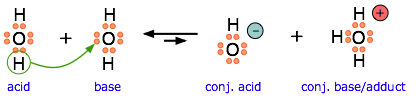Another example, showing the autoprotolysis of water. Note that the conjugate base is also the adduct.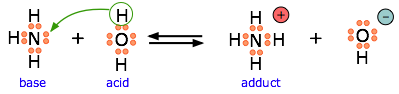Ammonia is both a Brønsted and a Lewis base, owing to the unshared electron pair on the nitrogen. The reverse of this reaction represents the hydrolysis of the ammonium ion.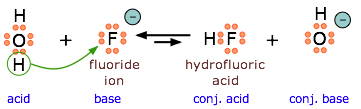Because HF is a weak acid, fluoride salts behave as bases in aqueous solution. As a Lewis base, F accepts a proton from water, which is transformed into a hydroxide ion.The bisulfite ion is amphiprotic and can act as an electron donor or acceptor.

Note

All Brønsted–Lowry bases (proton acceptors), such as OH, H2O, and NH3, are also electron-pair donors. Thus the Lewis definition of acids and bases does not contradict the Brønsted–Lowry definition. Rather, it expands the definition of acids to include substances other than the H+ ion.

## Lewis Acid-Base Neutralization without Transferring Protons

Electron-deficient molecules, such as BCl3, contain less than an octet of electrons around one atom and have a strong tendency to gain an additional pair of electrons by reacting with substances that possess a lone pair of electrons. Lewis’s definition, which is less restrictive than either the Brønsted–Lowry or the Arrhenius definition, grew out of his observation of this tendency. A general Brønsted–Lowry acid–base reaction can be depicted in Lewis electron symbols as follows:The proton (H+), which has no valence electrons, is a Lewis acid because it accepts a lone pair of electrons on the base to form a bond. The proton, however, is just one of many electron-deficient species that are known to react with bases. For example, neutral compounds of boron, aluminum, and the other Group 13 elements, which possess only six valence electrons, have a very strong tendency to gain an additional electron pair. Such compounds are therefore potent Lewis acids that react with an electron-pair donor such as ammonia to form an acid–base adduct, a new covalent bond, as shown here for boron trifluoride (BF3):The bond formed between a Lewis acid and a Lewis base is a coordinate covalent bond because both electrons are provided by only one of the atoms (N, in the case of F3B:NH3). After it is formed, however, a coordinate covalent bond behaves like any other covalent single bond.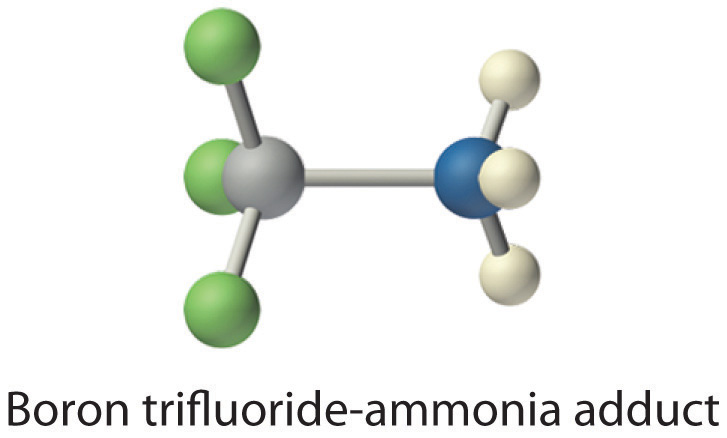Species that are very weak Brønsted–Lowry bases can be relatively strong Lewis bases. For example, many of the group 13 trihalides are highly soluble in ethers (R–O–R′) because the oxygen atom in the ether contains two lone pairs of electrons, just as in H2O. Hence the predominant species in solutions of electron-deficient trihalides in ether solvents is a Lewis acid–base adduct. A reaction of this type is shown in Figure $$\PageIndex{1}$$ for boron trichloride and diethyl ether:$$\textbf{Figure } \PageIndex{1}$$: Lewis Acid/Base reaction of boron trichloride and diethyl ether reaction

Note

• Electron-deficient molecules (those with less than an octet of electrons) are Lewis acids.
• The acid-base behavior of many compounds can be explained by their Lewis electron structures.

Many molecules with multiple bonds can act as Lewis acids. In these cases, the Lewis base typically donates a pair of electrons to form a bond to the central atom of the molecule, while a pair of electrons displaced from the multiple bond becomes a lone pair on a terminal atom.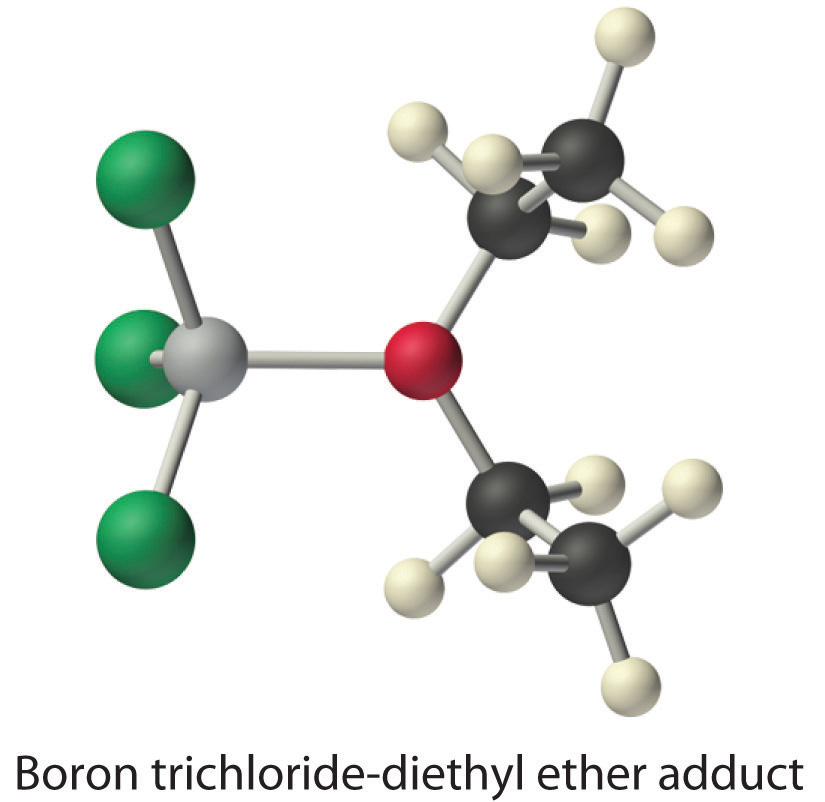Figure $$\PageIndex{2}$$. The highly electronegative oxygen atoms pull electron density away from carbon, so the carbon atom acts as a Lewis acid. Arrows indicate the direction of electron flow.$$\textbf{Figure } \PageIndex{2}$$: Lewis Acid/Base reaction of the hydroxide ion with carbon dioxide

$$\textbf{Example } \PageIndex{1}$$

Identify the acid and the base in each Lewis acid–base reaction.

1. BH3 + (CH3)2S → H3B:S(CH3)2
2. CaO + CO2 → CaCO3
3. BeCl2 + 2 Cl → BeCl42−

Given: reactants and products

Asked for: identity of Lewis acid and Lewis base

Strategy:

In each equation, identify the reactant that is electron deficient and the reactant that is an electron-pair donor. The electron-deficient compound is the Lewis acid, whereas the other is the Lewis base.

Solution:

1. In BH3, boron has only six valence electrons. It is therefore electron deficient and can accept a lone pair. Like oxygen, the sulfur atom in (CH3)2S has two lone pairs. Thus (CH3)2S donates an electron pair on sulfur to the boron atom of BH3. The Lewis base is (CH3)2S, and the Lewis acid is BH3.
2. As in the reaction shown in Equation 8.21, CO2 accepts a pair of electrons from the O2− ion in CaO to form the carbonate ion. The oxygen in CaO is an electron-pair donor, so CaO is the Lewis base. Carbon accepts a pair of electrons, so CO2 is the Lewis acid.
3. The chloride ion contains four lone pairs. In this reaction, each chloride ion donates one lone pair to BeCl2, which has only four electrons around Be. Thus the chloride ions are Lewis bases, and BeCl2 is the Lewis acid.

$$\textbf{Exercise } \PageIndex{1}$$

Identify the acid and the base in each Lewis acid–base reaction.

1. (CH3)2O + BF3 → (CH3)2O:BF3
2. H2O + SO3 → H2SO4

1. Lewis base: (CH3)2O; Lewis acid: BF3
2. Lewis base: H2O; Lewis acid: SO3

$$\textbf{Exercise } \PageIndex{2}$$

Here are several more examples of Lewis acid-base reactions that cannot be accommodated within the Brønsted or Arrhenius models. Identify the Lewis acid and Lewis base in each reaction.

1. $$Al(OH)_3 + OH^– \rightarrow Al(OH)_4^–$$
2. $$SnS_2 + S^{2–} \rightarrow SnS_3^{2–}$$
3. $$Cd(CN)_2 + 2 CN^– \rightarrow Cd(CN)_4^{2+}$$
4. $$AgCl + 2 NH_3 \rightarrow Ag(NH_3)_2^+ + Cl^–$$
5. $$Fe^{2+} + NO \rightarrow Fe(NO)^{2+}$$
6. $$[Ni^{2+} + 6 NH_3 \rightarrow Ni(NH_3)_5^{2+}$$

## Non-Aqueous Acid/Base Systems

We ordinarily think of Brønsted-Lowry acid-base reactions as taking place in aqueous solutions, but this need not always be the case. A more general view encompasses a variety of acid-base solvent systems, of which the water system is only one (Table $$\PageIndex{1}$$). Each of these has as its basis an amphiprotic solvent (one capable of undergoing autoprotolysis), in parallel with the familiar case of water.

The limiting acid in a given solvent is the solvonium ion, such as H3O+ (hydronium) ion in water. An acid which has more of a tendency to donate a hydrogen ion than the limiting acid will be a strong acid in the solvent considered, and will exist mostly or entirely in its dissociated form. Likewise, the limiting base in a given solvent is the solvate ion, such as OH (hydroxide) ion, in water. A base which has more affinity for protons than the limiting base cannot exist in solution, as it will react with the solvent.Figure } \PageIndex{3}\): Use of non-aqueous solvents allows the study of strong acids that are hindered by the "leveling effect" of the solvent.

The ammonia system is one of the most common non-aqueous system in Chemistry. Liquid ammonia boils at –33° C, and can conveniently be maintained as a liquid by cooling with dry ice (–77° C). It is a good solvent for substances that also dissolve in water, such as ionic salts and organic compounds since it is capable of forming hydrogen bonds. Bases can exist in solution in liquid ammonia which cannot exist in aqueous solution: this is the case for any base which is stronger than the hydroxide ion, but weaker than the amide ion $$NH_2^-$$. The limiting acid in liquid ammonia is the ammonium ion, which has a pKa value in water of 9.25. The limiting base is the amide ion, NH2. This is a stronger base than the hydroxide ion and so cannot exist in aqueous solution. The pKa value of ammonia is estimated to be approximately 33. Any acid which is a stronger acid than the ammonium ion will be a strong acid in liquid ammonia. This is the case for acetic acid, which is completely dissociated in liquid ammonia solution. The addition of pure acetic acid and the addition of ammonium acetate have exactly the same effect on a liquid ammonia solution: the increase in its acidity: in practice, the latter is preferred for safety reasons.

Note

As with $$OH^-$$ and $$H_3O^+$$ in water, the strongest acid and base in $$NH_3$$ is dictated by the corresponding autoprotolysis reaction of the solvent:

$2 NH_3 \rightleftharpoons NH_4^+ + NH_2^– \nonumber$

One use of non-aqueous acid-base systems is to examine the relative strengths of the strong acids and bases, whose strengths are "leveled" by the fact that they are all totally converted into H3O+ or OH ions in water. By studying them in appropriate non-aqueous solvents which are poorer acceptors or donors of protons, their relative strengths can be determined. Many familiar substances can serve as the basis of protonic solvent systems (Table $$\PageIndex{1}$$).

 solvent autoprotolysis reaction pKa water $$2 H_2O \rightleftharpoons H_3O^+ + OH^–$$ 14 ammonia $$2 NH_3 \rightleftharpoons NH_4^+ + NH_2^–$$ 33 acetic acid $$2 CH_3COOH \rightleftharpoons CH_3COOH_2^+ + CH_3COO^–$$ 13 ethanol $$2 C_2H_5OH \rightleftharpoons C_2H_5OH_2^+ + C_2H_5O^–$$ 19 hydrogen peroxide $$2 HO-OH \rightleftharpoons HO-OH_2^+ + HO-O^–$$ 13 hydrofluoric acid $$2 HF \rightleftharpoons H^2F^+ + F^–$$ 10 sulfuric acid $$2 H_2SO_4 \rightleftharpoons H3SO_4^+ + HSO_4^–$$ 3.5

The extreme case is a superacid, a medium in which the hydrogen ion is only very weakly solvated. The classic example is a mixture of antimony pentafluoride and liquid hydrogen fluoride:

$SbF_5 + HF \rightleftharpoons H^+ + SbF_6^−$

The limiting base, the hexfluoroantimonate anion $$SbF_6^−$$, is so weakly attracted to the hydrogen ion that virtually any other base will bind more strongly: hence, this mixture can be used to protonate organic molecules which would not be considered bases in other solvents. It should noted that pH is undefined in aprotic solvents, which assumes presence of hydronium ions. In other solvents, the concentration of the respective solvonium/solvate ions should be used (e.g., $$[NH_4^+]$$ and $$[NH_2^–]$$ in $$NH_{3(l)}$$.